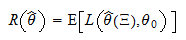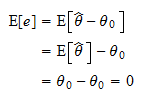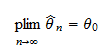StatLect

# Point estimation

In the lecture entitled Statistical inference we have defined statistical inference as the act of using a sample to make statements about the probability distribution that generated the sample. The sampleis regarded as the realization of a random vector, whose unknown joint distribution function, denoted by, is assumed to belong to a set of distribution functions, called statistical model (or, simply, model).

When the modelis put into correspondence with a setof real vectors, then we have a parametric model.is called the parameter space and its elements are called parameters. Denote bythe parameter that is associated with the unknown distribution functionand assume thatis unique.is called the true parameter, because it is associated to the distribution that actually generated the sample. This lecture introduces a type of inference about the true parameter called point estimation.## Estimate and estimator

Roughly speaking, point estimation is the act of choosing a parameterwhich is our best guess of of the true (and unknown) parameter. Our best guessis called an estimate of.

When the estimateis produced using a predefined rule (a function) that associates a parameter estimateto eachin the support of, we can writeThe functionis called an estimator. Often, the symbolis used to denote both the estimate and the estimator. The meaning is usually clear from the context.

## Estimation error, loss and risk

Using the decision-theoretic terminology introduced in the lecture entitled Statistical inference, making an estimateis an act, which produces consequences. Among the consequences that are usually considered in a parametric decision problem, the most relevant one is the estimation error.

The estimation erroris the difference between the estimateand the true parameter:Of course, the statistician's goal is to commit the smallest possible estimation error. This preference can be formalized using loss functions. A loss function, mappinginto, quantifies the loss incurred by estimatingwith.

Frequently used loss functions are:

1. the absolute error:whereis the Euclidean norm (it coincides with the absolute value when);

2. the squared error:When the estimateis obtained from an estimator (a function of the sample, which in turn is a realization of the random vector), then the losscan be thought of as a random variable. Its expected value is called the statistical risk (or, simply, the risk) of an estimatorand it is denoted by:Note that the expected value in the above definition of risk is computed with respect to the true distribution function. Therefore, in order to compute the risk, we need to know not only the true parameter, but also the distribution function of(i.e.). In practice, the risk in never known, becauseandare unknown, so also the risk needs to be estimated. For example, we can compute an estimateof the risk by pretending that the estimatewere the true parameter, denoting bythe estimator ofand computing the estimated risk aswhere the expected value is with respect to the estimated distribution function.

Even if the risk is unknown, the notion of risk is often used to derive theoretical properties of estimators. In any case, parameter estimation is always guided, at least ideally, by the principle of risk minimization, i.e. by the search for estimatorsthat minimize the risk.

Depending on the specific loss function we use, the statistical risk of an estimator can take different names:

1. when the absolute error is used as a loss function, then the riskis called the mean absolute error of the estimator.

2. when the squared error is used as a loss function, then the riskis called mean squared error (MSE). The square root of the mean squared error is called root mean squared error (RMSE).

## Other criteria to evaluate estimators

In this section we discuss other criteria that are commonly used to evaluate estimators.

### Unbiasedness

If an estimator produces parameter estimates that are on average correct, then it is said to be unbiased. The following is a formal definition:

Definition Letbe the true parameter and letbe an estimator of.is an unbiased estimator ofif and only ifIf an estimator is not unbiased, then it is called a biased estimator.

Note that in the above definition of unbiasednessis a shorthand forwhereis the random vector of which the sampleis a realization and the expected value is computed with respect to the true distribution function.

Also note that if an estimator is unbiased, this implies that the estimation error is on average zero:### Consistency

If an estimator produces parameter estimates that converge to the true value when the sample size increases, then it is said to be consistent. The following is a formal definition.

Definition Letbe a sequence of samples such that all the distribution functionsare put into correspondence with the same parameter. A sequence of estimatorsis said to be consistent (or weakly consistent) if and only ifwhereindicates convergence in probability. The sequence of estimators is said to be strongly consistent if and only ifwhereindicates almost sure convergence. A sequence of estimators which is not consistent is called inconsistent.

When the sequence of estimators is obtained using the same predefined rule for every sample, we often say, with a slight abuse of language, "consistent estimator" instead of saying "consistent sequence of estimators". In such cases, what we mean is that the predefined rule produces a consistent sequence of estimators.

## Examples

You can find examples of point estimation in the lectures entitled Point estimation of the mean and Point estimation of the variance.

The book

Most of the learning materials found on this website are now available in a traditional textbook format.

Glossary entries
Share